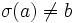# Conjugacy-closed normal not implies central factor

(diff) ← Older revision | Latest revision (diff) | Newer revision → (diff)
This article gives the statement and possibly, proof, of a non-implication relation between two subgroup properties. That is, it states that every subgroup satisfying the first subgroup property (i.e., conjugacy-closed normal subgroup) need not satisfy the second subgroup property (i.e., central factor)
View a complete list of subgroup property non-implications | View a complete list of subgroup property implications
Get more facts about conjugacy-closed normal subgroup|Get more facts about central factor
EXPLORE EXAMPLES YOURSELF: View examples of subgroups satisfying property conjugacy-closed normal subgroup but not central factor|View examples of subgroups satisfying property conjugacy-closed normal subgroup and central factor

## Statement

### Property-theoretic statement

The subgroup property of being a conjugacy-closed normal subgroup is not stronger than the subgroup property of being a central factor.

## Definitions used

### Conjugacy-closed normal subgroup

Further information: Conjugacy-closed normal subgroup

• Hands-on definition: A subgroup$H$ of a group$G$ is termed a conjugacy-closed normal subgroup if$H$ is normal in$G$, and, whenever two elements of$H$ are conjugate in$G$, they are conjugate in$H$.
• Definition in terms of function restriction expressions: The property of being conjugacy-closed normal can be expressed using the following function restriction expression:

Inner automorphism$\to$ Class-preserving automorphism

In other words,$H$ is conjugacy-closed normal in$G$ if every inner automorphism of$G$ restricts to a class-preserving automorphism of$H$ -- an automorphism that preserves conjugacy classes.

### Central factor

Further information: Central factor

• Hands-on definition: A subgroup$H$ of a group$G$ is termed a central factor if$HC_G(H) = G$.
• Definition in terms of function restriction expressions: The property of being a central factor can be expressed as:

Inner automorphism$\to$ Inner automorphism

In other words,$H$ is a central factor in$G$ if every inner automorphism of$G$ restricts to an inner automorphism of$H$.

## Facts used

1. Class-preserving not implies inner

## Proof

### Proof using fact (1)

We shall prove the following. If$H$ possesses a class-preserving automorphism$\sigma$ that is not inner, we can find a group$G$ containing$H$ as a conjugacy-closed normal subgroup that is not a central factor.

Let$K$ be the cyclic subgroup of$\operatorname{Aut}(H)$ generated by$\sigma$, and let$G = H \rtimes K$, with the specified action of$K$ on$H$.

•$H$ is conjugacy-closed normal in$G$: Indeed, any inner automorphism of$G$ is generated by$\sigma$ and inner automorphisms from$H$, and each of these restrict to class-preserving automorphisms of$H$.
•$H$ is not a central factor of$G$: Indeed, conjugation by the element$\sigma \in G$ induces the automorphism$\sigma$ on$H$, that is not an inner automorphism.

### An infinite group example

In the symmetric group$S(\Omega)$ on an infinite set$\Omega$, the subgroup$FS(\Omega)$ comprising the finitary permutations (those permutations that move only finitely many elements) is a conjugacy-closed subgroup: two finitary permutations that are conjugate by some permutation are conjugate by a finitary permutation. Further, it is a normal subgroup: any conjugate to a finitary permutation is again finitary.

Thus, the finitary symmetric group$FS(\Omega)$ is a conjugacy-closed normal subgroup in the whole symmetric group$S(\Omega)$. On the other hand, it is not a central factor. To see this, observe that by the first definition of central factor, it suffices to show that the centralizer in the whole symmetric group, of the finitary symmetric group, is trivial.

Let's do this. Suppose$\sigma \in S(\Omega)$ commutes with every element in$FS(\Omega)$. Now, pick$a \in \Omega$. We will show that$\sigma(a) = a$.

First, since$\Omega$ has at least three elements, we can pick$b \in \Omega$ such that$\sigma(a) \ne b, a \ne b$. Let$\tau = (a,b)$ be a transposition.

Since$\sigma$ commutes with$\tau$, we are forced to conclude that either$\sigma(a) = b$ or$\sigma(a) = a$. Since$\sigma(a) \ne b$ by assumption,$\sigma(a) = a$, completing the proof.